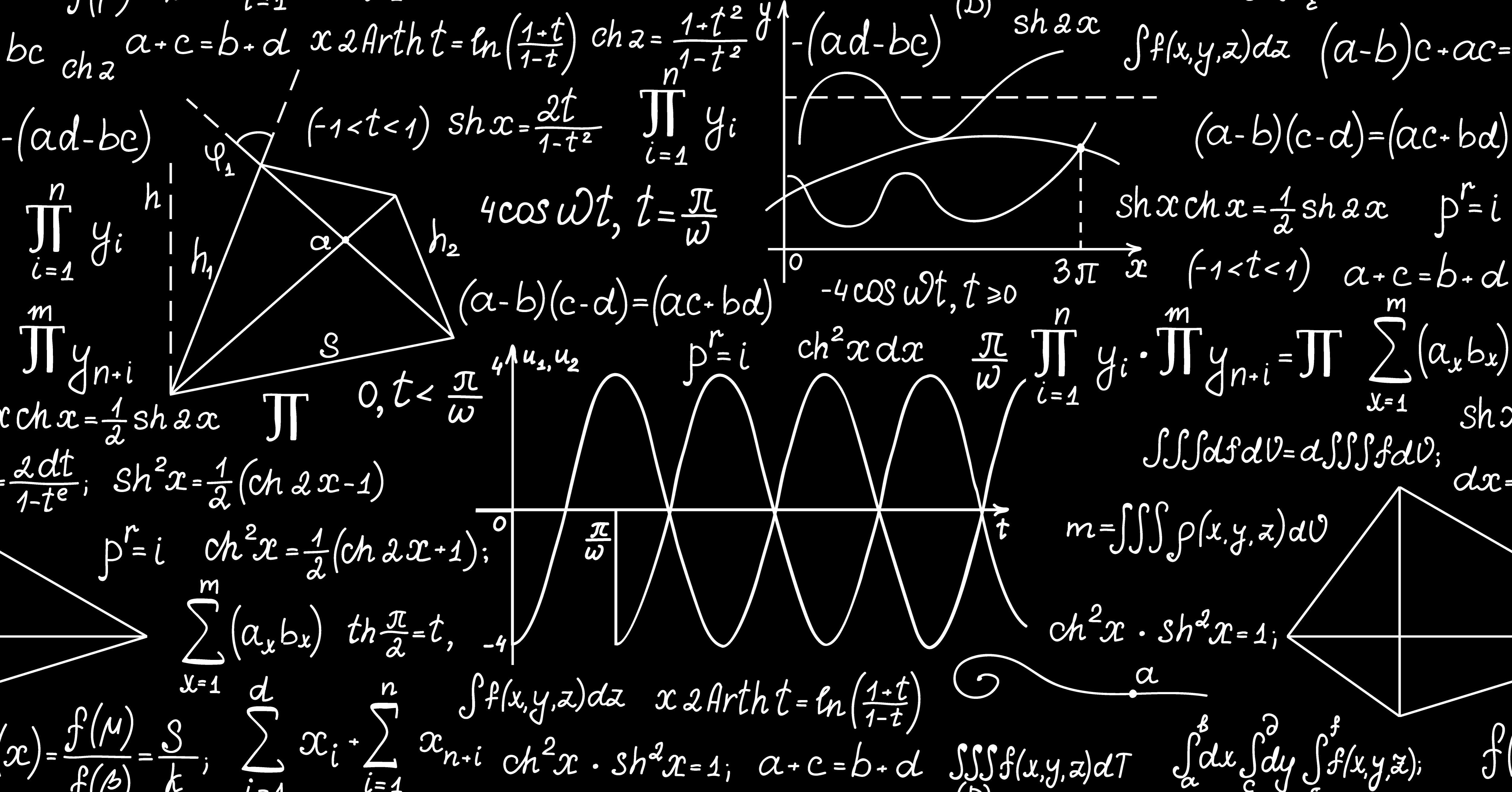Homework
Share
Explore# Unit 1.9.1

EQ: What are Perfect Squares and cubes and how do you calculate the square root of numbers that are not Perfect Squares?
Perfect Square: A perfect square is a number that can be made by multiplying an integer by itself.
Square Root: A square root is one of two equal factors of a number.
When solving a square root, think to yourself - what number times itself equals the number under the radical?
Keep in mind that all numbers could have a positive and negative square root.
Perfect Cube: A perfect cube is the product of three equal integers. (For example, 8 is a perfect cube because 2 x 2 x 2 = 8)
Cube Root: The cube root of a number is a special value that, when used in a multiplication three times, gives that number. (For example, 3 is the cube root of 27 because 3 x 3 x 3 = 27)
Practice:
Digital Worksheet Practice:
Assignment: